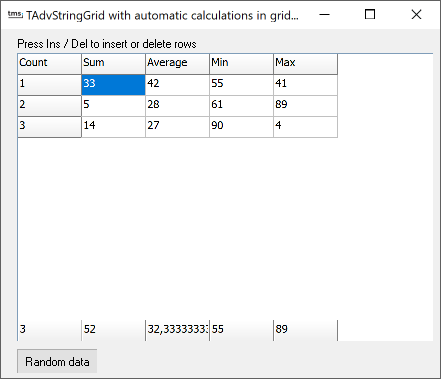### Example 49 : auto calculating floating footersIt is now very convenient to show automatically calculated values in the fixed floating footer of TAdvStringGrid. Automatic calculations of column sum, column minimum, column maximum, column average and column rowcount can be easily added. Only 2 steps are required to enable this :
```grid.FloatingFooter.Visible := True; // show the floating footer
grid.FloatingFooter.ColumnCalc[colindex] := CalcType; // set the calculation type for the column colindex in the floating footer.
```
The calculation type CalcType can be:

acCount: show rowcount
acSum: show column sum
acAvg: show column average
acMin: show column minimum
acMax: show column maximum

As such, the sample app's setup was done with:

```procedure TForm1.FormCreate(Sender: TObject);
begin